# Palindromic Tree | Introduction & Implementation

• Difficulty Level : Hard
• Last Updated : 24 May, 2019

We encounter various problems like Maximum length palindrome in a string, number of palindromic substrings and many more interesting problems on palindromic substrings . Mostly of these palindromic substring problems have some DP O(n2) solution (n is length of the given string) or then we have a complex algorithm like Manacher’s algorithm which solves the Palindromic problems in linear time.

In this article, we will study an interesting Data Structure, which will solve all the above similar problems in much more simpler way. This data structure is invented by Mikhail Rubinchik.

```Features of Palindromic Tree : Online query and updation
Easy to implement
Very Fast```

Structure of Palindromic Tree

Palindromic Tree’s actual structure is close to directed graph. It is actually a merged structure of two Trees which share some common nodes(see the figure below for better understanding). Tree nodes store palindromic substrings of given string by storing their indices.
This tree consists of two types of edges :
1) Insertion edge (weighted edge)
2) Maximum Palindromic Suffix (un-weighted)

Insertion Edge :
Insertion edge from a node u to v with some weight x means that the node v is formed by inserting x at the front and end of the string at u. As u is already a palindrome, hence the resulting string at node v will also be a palindrome.
x will be a single character for every edge. Therefore, a node can have max 26 insertion edges (considering lower letter string). We will use orange color for this edge in our pictorial representation.

Maximum Palindromic Suffix Edge:
As the name itself indicates that for a node this edge will point to its Maximum Palindromic Suffix String node. We will not be considering the complete string itself as the Maximum Palindromic Suffix as this will make no sense(self loops). For simplicity purpose, we will call it as Suffix edge(by which we mean maximum suffix except the complete string). It is quite obvious that every node will have only 1 Suffix Edge as we will not store duplicate strings in the tree. We will use Blue dashed edges for its Pictorial representation.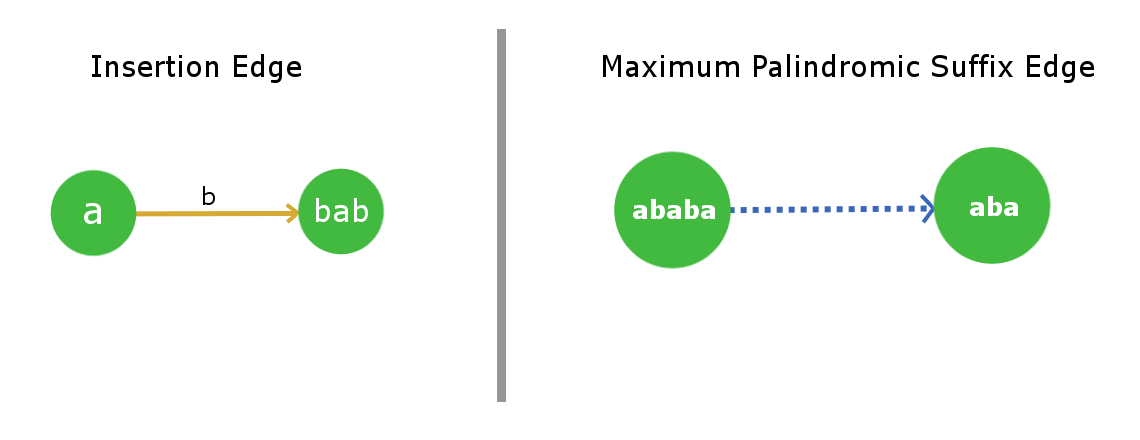Root Nodes and their convention:
This tree/graph data structure will contain 2 root dummy nodes. More, precisely consider it as roots of two separate trees, which are linked together.
Root-1 will be a dummy node which will describe a string for length = -1 ( you can easily infer from the implementation point of view that why we used so ). Root-2 will be a node which will describe a null string of length = 0.

Root-1 has a suffix edge connected to itself(self-loop) as for any imaginary string of length -1 , its Maximum palindromic suffix will also be imaginary, so this is justified. Now Root-2 will also have its suffix edge connected to Root-1 as for a null string (length 0) there is no real palindromic suffix string of length less than 0.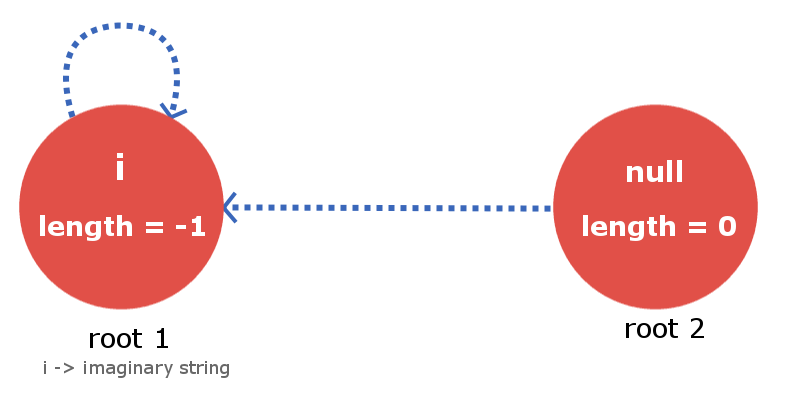Building the Palindromic Tree

To build a Palindromic Tree, we will simply insert the characters in our string one by one till we reach its end and when we are done inserting we will be with our palindromic tree which will contain all the distinct palindromic substrings of the given strings. All we need to ensure is that, at every insertion of a new character, our palindromic tree maintains the above discussed feature. Let’s see how we can accomplish it.

Let’s say we are given a string s with length l and we have inserted the string up till index k (k < l-1). Now, we need to insert the (k+1)th character. Insertion of (k+1)th character means insertion of a node that is longest palindrome ending at index (k+1). So, the longest palindromic string will be of form ( ‘s[k+1]’ + “X” + ‘s[k+1]’ ) and X will itself be a palindrome.Now the fact is that the string X lies at index < k+1 and is palindrome. So, it will already exist in our palindromic tree as we have maintained the very basic property of it saying that it will contain all the distinct palindromic substrings.

So, to insert the character s[k+1] ,we only need to find the String X in our tree and direct the insertion edge from X with weight s[k+1] to a new node , which contains s[k+1]+X+s[k+1]. The main job now is to find the string X in efficient time. As we know that we are storing the suffix link for all the nodes. Therefore to track the node with string X we just need to move down the suffix link for the current node i.e the node which contains insertion of s[k]. See the below image for better understanding.

The current node in the below figure tells that it is the largest palindrome that ends at index k after processing all the indices from 0 to k. The blue dotted path is the link of suffix edges from current node to other processed nodes in the tree. String X will exist in one of these nodes that lie on this chain of suffix link. All we need is to find it by iterating over it down the chain.

To find the required node that contains the string X we will place the k+1 th character at the end of every node that lies in suffix link chain and check if first character of the corresponding suffix link string is equal to the k+1 th character.

Once, we find the X string we will direct an insertion edge with weight s[k+1] and link it to the new node that contains largest palindrome ending at index k+1. The array elements between the brackets as described in the figure below are the nodes that are stored in the tree.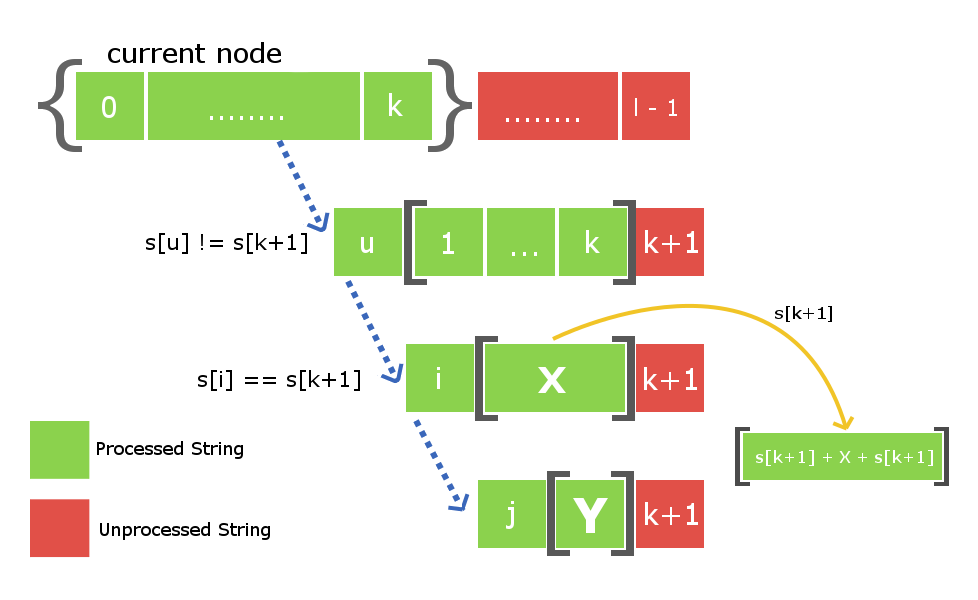There is one more thing left that is to be done. As we have created a new node at this s[k+1] insertion , therefore we will also have to connect it with its suffix link child. Once, again to do so we will use the above down the suffix link iteration from node X to find some new string Y such that s[k+1] + Y + s[k+1] is a largest palindromic suffix for the newly created node. Once, we find it we will then connect the suffix link of our newly created node with the node Y.

Note : There are two possibilities when we find the string X. First possibility is that string s[k+1]Xs[k+1] do not exist in the tree and second possibility is if it already exists in the tree. In first case we will proceed the same way but in second case we will not create a new node separately but will just link the insertion edge from X to already existing S[k+1]+X+S[k+1] node in the tree. We also need not to add the suffix link because the node will already contain its suffix link.

Consider a string s = “abba” with length = 4.

At initial state we will have our two dummy root nodes one with length -1 ( some imaginary string i ) and second a null string with length 0. At this point we haven’t inserted any character in the tree. Root1 i.e root node with length -1 will be the current node from which insertion takes place.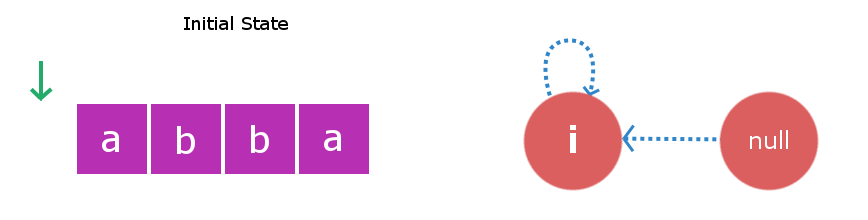Stage 1: We will insert s i.e ‘a‘.We will start checking from the current node i.e Root1. Inserting ‘a’ at start and end of a string with length -1 will yield to a string with length 1 and this string will be “a”. Therefore, we create a new node “a” and direct insertion edge from root1 to this new node. Now, largest palindromic suffix string for string of length 1 will be a null string so its suffix link will be directed to root2 i.e null string.Now the current node will be this new node “a”.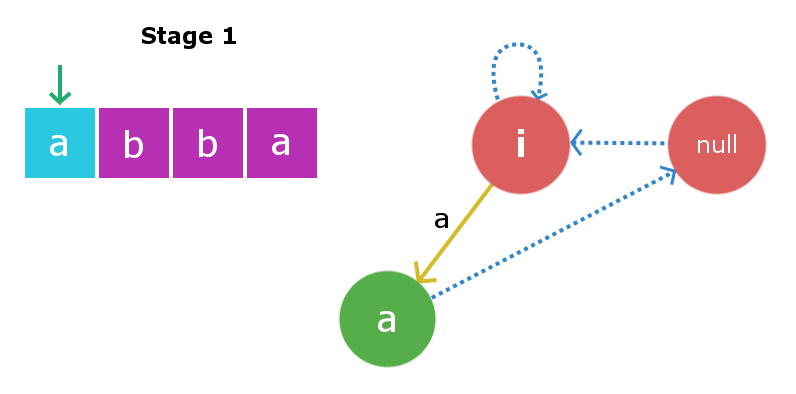Stage 2: We will insert s i.e ‘b‘. Insertion process will start from current node i.e “a” node. We will traverse the suffix link chain starting from current node till we find suitable X string , So here traversing the suffix link we again found root1 as X string. Once again inserting ‘b’ to string of length -1 will yield a string of length 1 i.e string “b”. Suffix link for this node will go to null string as described in above insertion. Now the current node will be this new node “b”.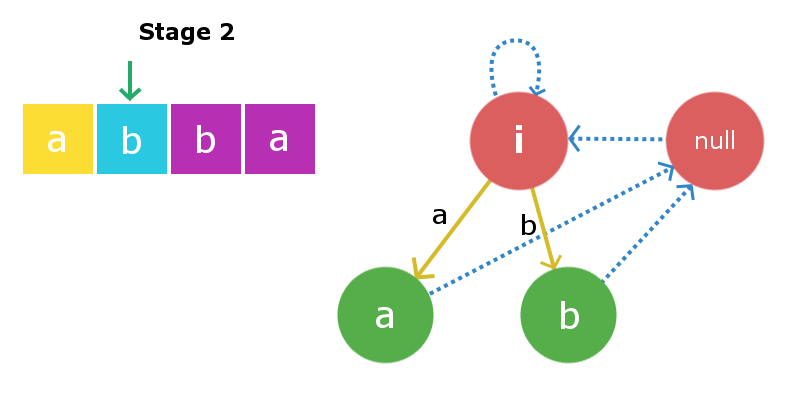Stage 3: We will insert s i.e ‘b‘. Once again starting from current node we will traverse its suffix link to find required X string. In this case it founds to be root2 i.e null string as adding ‘b’ at front and end of null string yields a palindrome “bb” of length 2. Therefore, we will create a new node “bb” and will direct the insertion edge from the null string to the newly created string. Now, the largest suffix palindrome for this current node will be node “b”. So, we will link the suffix edge from this newly created node to node “b”. Current node now becomes node “bb”.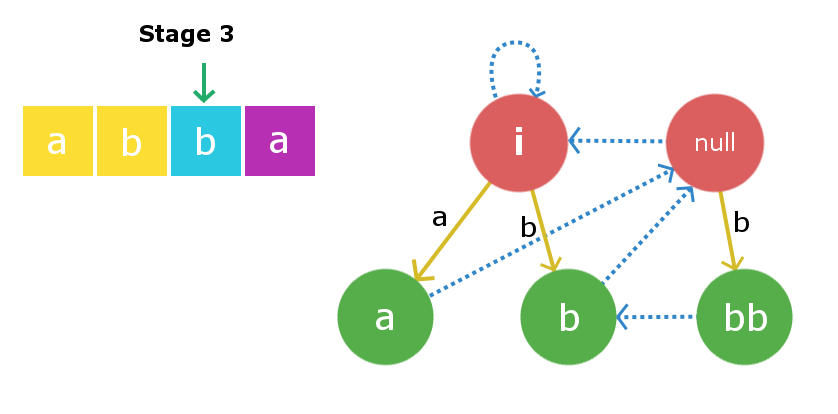Stage 4: We will insert s i.e ‘a‘. Insertion process begins with current node and in this case the current node itself is the largest X string such that s + X + s is palindrome. Therefore, we will create a new node “abba” and link the insertion edge from the current node “bb” to this newly created node with edge weight ‘a’. Now, the suffix the link from this newly created node will be linked to node “a” as that is the largest palindromic suffix.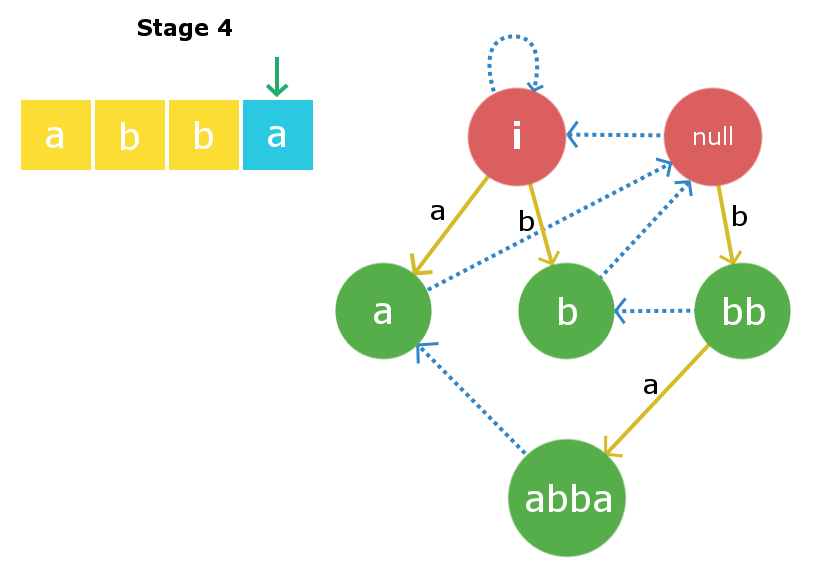The C++ implementation for the above implementation is given below :

## Recommended: Please try your approach on {IDE} first, before moving on to the solution.

 `// C++ program to demonstrate working of``// palindromic tree``#include "bits/stdc++.h"``using` `namespace` `std;`` ` `#define MAXN  1000`` ` `struct` `Node``{``    ``// store start and end indexes of current``    ``// Node inclusively``    ``int` `start, end;`` ` `    ``// stores length of substring``    ``int` `length;`` ` `    ``// stores insertion Node for all characters a-z``    ``int` `insertEdg;`` ` `    ``// stores the Maximum Palindromic Suffix Node for``    ``// the current Node``    ``int` `suffixEdg;``};`` ` `// two special dummy Nodes as explained above``Node root1, root2;`` ` `// stores Node information for constant time access``Node tree[MAXN];`` ` `// Keeps track the current Node while insertion``int` `currNode;``string s;``int` `ptr;`` ` `void` `insert(``int` `idx)``{``//STEP 1//`` ` `    ``/* search for Node X such that s[idx] X S[idx]``       ``is maximum palindrome ending at position idx``       ``iterate down the suffix link of currNode to``       ``find X */``    ``int` `tmp = currNode;``    ``while` `(``true``)``    ``{``        ``int` `curLength = tree[tmp].length;``        ``if` `(idx - curLength >= 1 and s[idx] == s[idx-curLength-1])``            ``break``;``        ``tmp = tree[tmp].suffixEdg;``    ``}`` ` `    ``/* Now we have found X ....``     ``* X = string at Node tmp``     ``* Check : if s[idx] X s[idx] already exists or not*/``    ``if``(tree[tmp].insertEdg[s[idx]-``'a'``] != 0)``    ``{``        ``// s[idx] X s[idx] already exists in the tree``        ``currNode = tree[tmp].insertEdg[s[idx]-``'a'``];``        ``return``;``    ``}`` ` `    ``// creating new Node``    ``ptr++;`` ` `    ``// making new Node as child of X with``    ``// weight as s[idx]``    ``tree[tmp].insertEdg[s[idx]-``'a'``] = ptr;`` ` `    ``// calculating length of new Node``    ``tree[ptr].length = tree[tmp].length + 2;`` ` `    ``// updating end point for new Node``    ``tree[ptr].end = idx;`` ` `    ``// updating the start for new Node``    ``tree[ptr].start = idx - tree[ptr].length + 1;`` ` ` ` `//STEP 2//`` ` `    ``/* Setting the suffix edge for the newly created``       ``Node tree[ptr]. Finding some String Y such that``       ``s[idx] + Y + s[idx] is longest possible``       ``palindromic suffix for newly created Node.*/`` ` `    ``tmp = tree[tmp].suffixEdg;`` ` `    ``// making new Node as current Node``    ``currNode = ptr;``    ``if` `(tree[currNode].length == 1)``    ``{``        ``// if new palindrome's length is 1``        ``// making its suffix link to be null string``        ``tree[currNode].suffixEdg = 2;``        ``return``;``    ``}``    ``while` `(``true``)``    ``{``        ``int` `curLength = tree[tmp].length;``        ``if` `(idx-curLength >= 1 and s[idx] == s[idx-curLength-1])``            ``break``;``        ``tmp = tree[tmp].suffixEdg;``    ``}`` ` `    ``// Now we have found string Y``    ``// linking current Nodes suffix link with s[idx]+Y+s[idx]``    ``tree[currNode].suffixEdg = tree[tmp].insertEdg[s[idx]-``'a'``];``}`` ` `// driver program``int` `main()``{``    ``// initializing the tree``    ``root1.length = -1;``    ``root1.suffixEdg = 1;``    ``root2.length = 0;``    ``root2.suffixEdg = 1;`` ` `    ``tree = root1;``    ``tree = root2;``    ``ptr = 2;``    ``currNode = 1;`` ` `    ``// given string``    ``s = ``"abcbab"``;``    ``int` `l = s.length();`` ` `    ``for` `(``int` `i=0; i

Output:

```All distinct palindromic substring for abcbab :
1)a
2)b
3)c
4)bcb
5)abcba
6)bab
```

Time Complexity
The time complexity for the building process will be O(k*n), here “n” is the length of the string and ‘k‘ is the extra iterations required to find the string X and string Y in the suffix links every time we insert a character.Let’s try to approximate the constant ‘k’. We shall consider a worst case like s = “aaaaaabcccccccdeeeeeeeeef”. In this case for similar streak of continuous characters it will take extra 2 iterations per index to find both string X and Y in the suffix links , but as soon as it reaches some index i such that s[i]!=s[i-1] the left most pointer for the maximum length suffix will reach its rightmost limit. Therefore, for all i when s[i]!=s[i-1] , it will cost in total n iterations(summing over each iteration) and for rest i when s[i]==s[i-1] it takes 2 iteration which sums up over all such i and takes 2*n iterations. Hence, approximately our complexity in this case will be O(3*n) ~ O(n).So, we can roughly say that the constant factor ‘k’ will be very less. Therefore, we can consider the overall complexity to be linear O(length of string). You may refer the reference links for better understanding.

References :

This article is contributed by Nitish Kumar. If you like GeeksforGeeks and would like to contribute, you can also write an article using contribute.geeksforgeeks.org or mail your article to contribute@geeksforgeeks.org. See your article appearing on the GeeksforGeeks main page and help other Geeks.